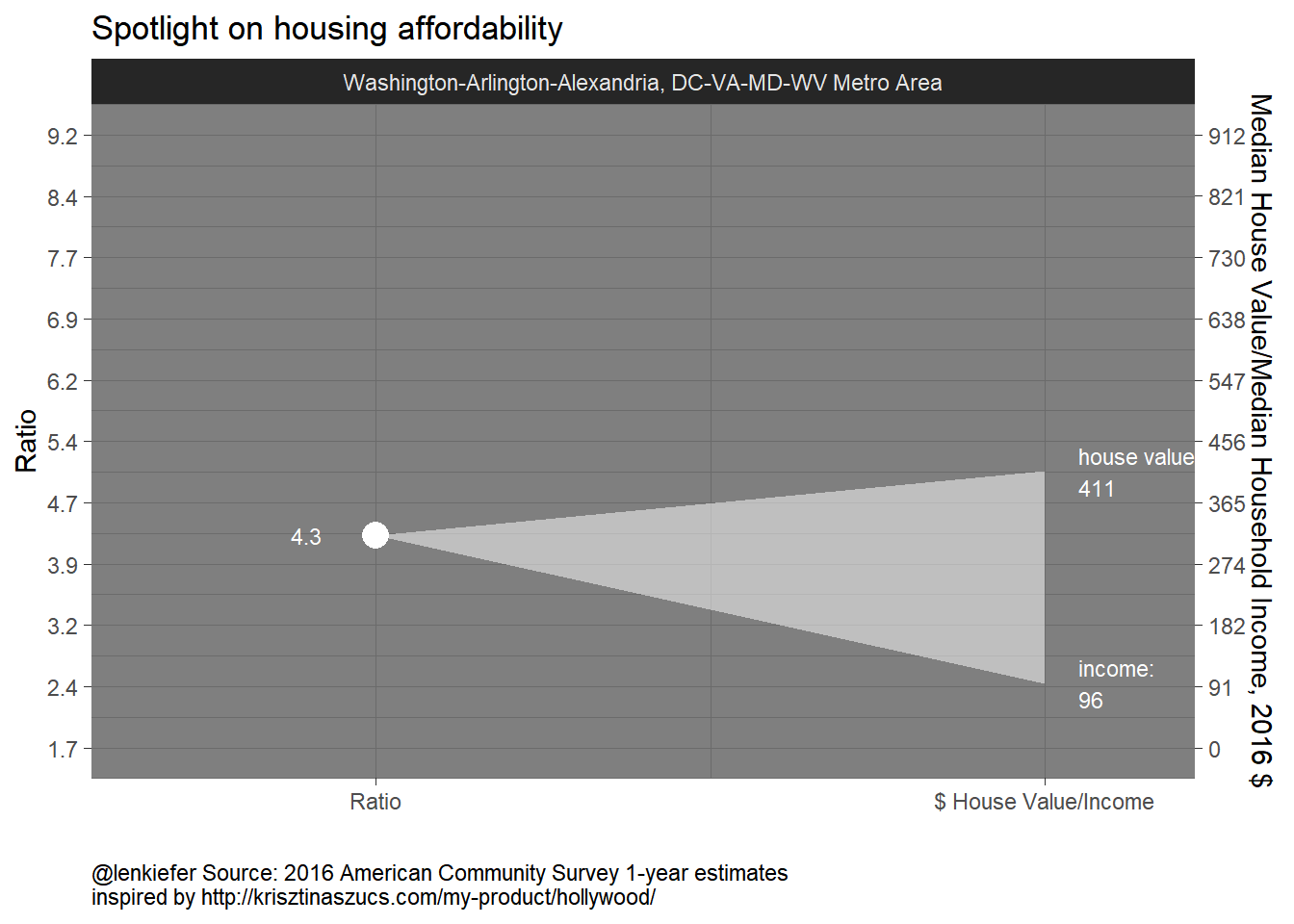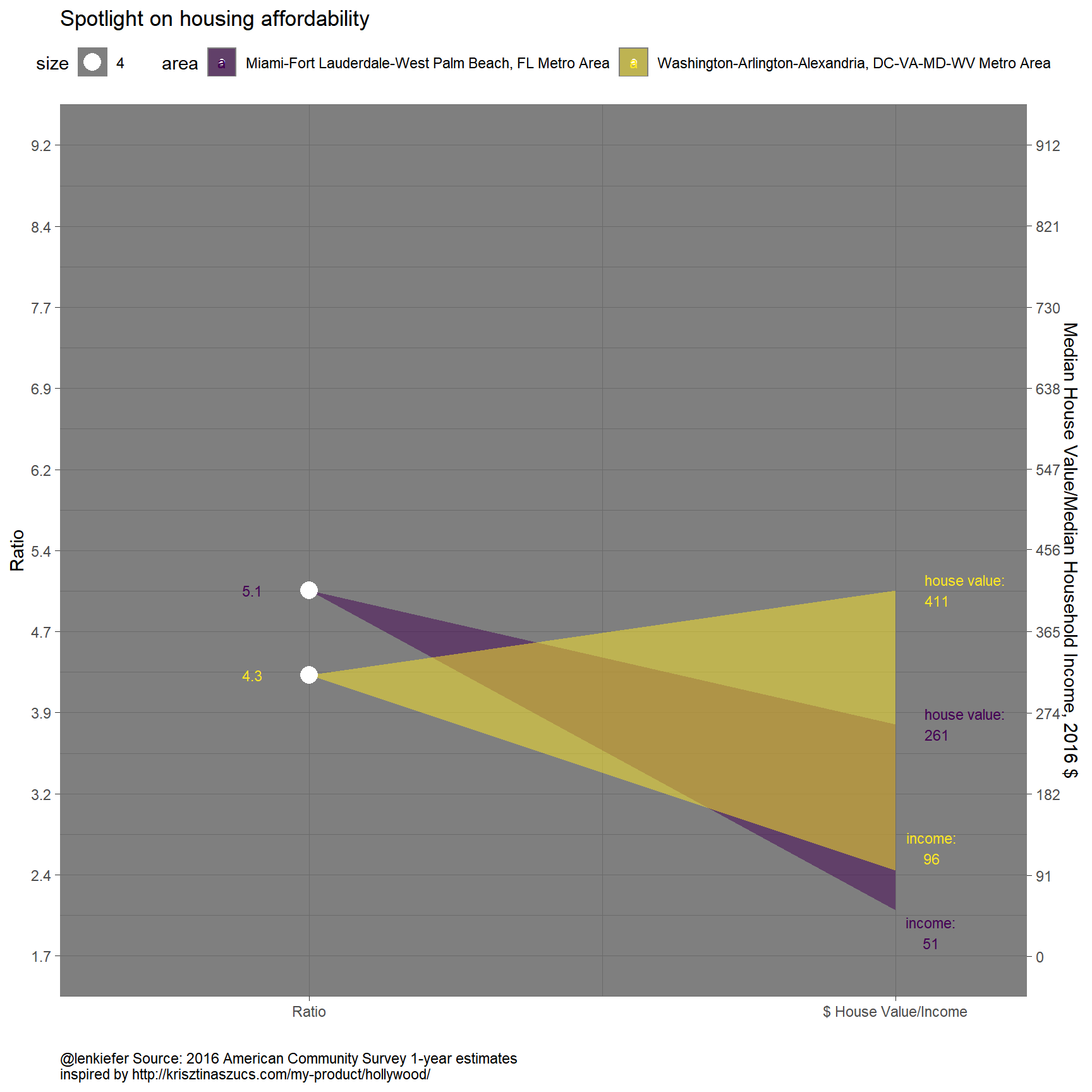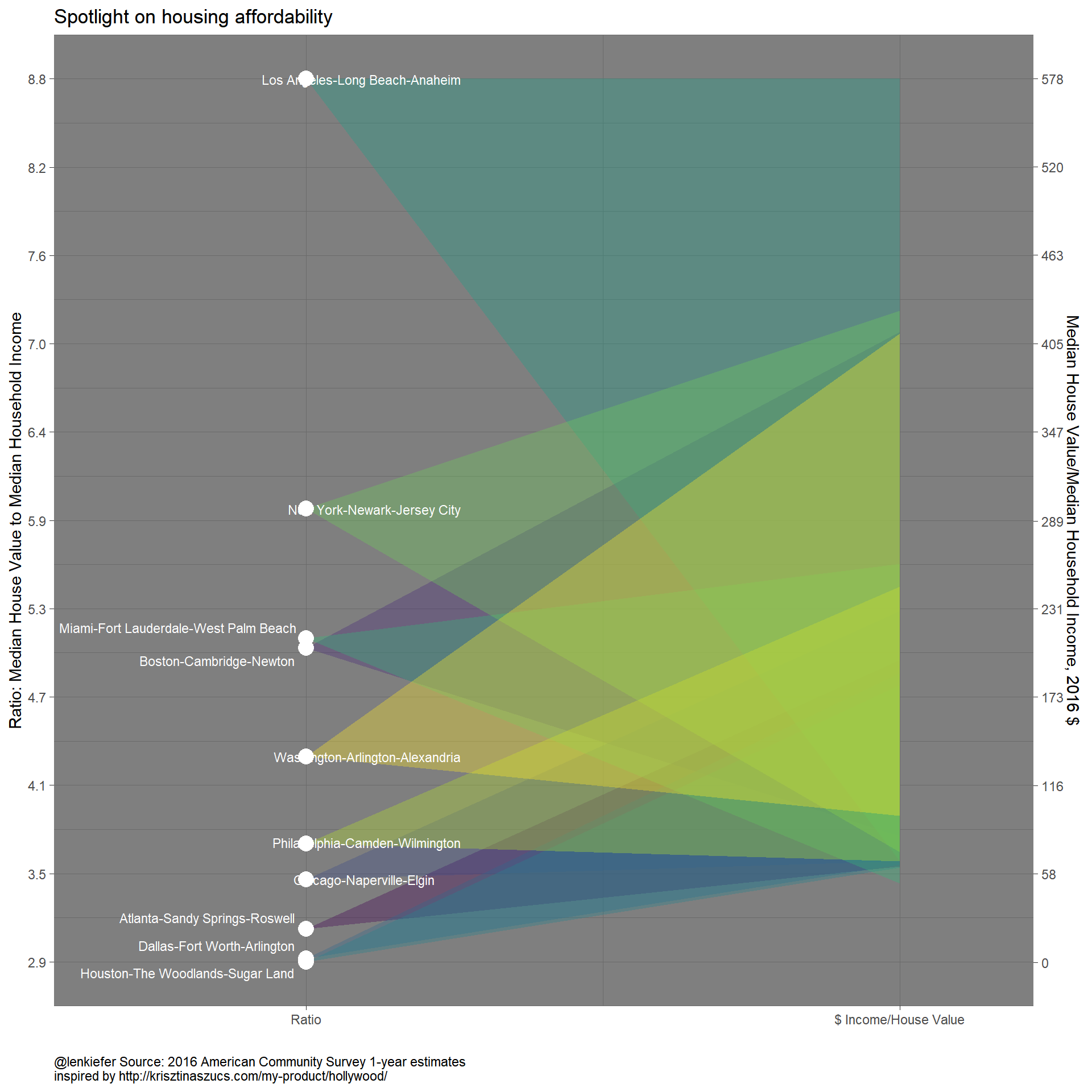# Spotlight on housing affordability

## Friends don't let freinds use dual y axis charts, but this might be an exception

IN MY LINE OF WORK, (finance/economics) you see a lot of dual axis line charts. I am of the opinion that dual y axis charts are sort of evil. But in this post I’m going to make one. It’s for a totally legit reason though.

Like in an earlier post we’ll make a graph similar to one I saw on xenographics. Xenographics gives examples of and links to “weird, but (sometimes) useful charts”. The examples xenographics gives are undoubtedly interesting and might help inspire you if you’re looking for something new.

Per usual, let’s make a graph with R.

# Housing affordability

I’m scheduled to give a talk on house price trends. During my talk I’m going to compare recent house price trends to income. For recent trends see this tweet thread.

For my upcoming talk I want a special kind of graphic to compare house prices to income. After looking around xenographics I decided that spotlight charts might be a good visual for my talk. See here for the original dataviz.

But in order to make this chart with R we’re going to need to use a dual axis. Fortunately in recent versions of ggplot2 ther’es an ability to add a secondary y axis despite potential issues with them. See for example, this post by DataWrapper’s Lisa Charlotte Rost on Twitter that looks at these charts.

# The plan

We’re going to compare median household income to median house prices by metro area. We’ll use a spotlight graph to expand the ratio by metro into its numerator and denominator. The ratio will serve as the light source, while the numerator and denominator will define the cone extending from the ratio.

## Get data

We will use the acs packaged to access the U.S. Census Bureau’s American Community Survey (ACS) API and get data. Note for this to work for you you’ll need to get an API key from the U.S. Census Bureau.

The latest data from the ACS is for 2016 (in my talk I’ll use more recent data from other sources). Let’s access the 1-year estimates and gather data on metropolitan stastical area (MSA) median household income and median house values. For other ways to visualize these kinds of data see this post from last year.

#####################################################################################
#####################################################################################
library(acs)
library(scales)
library(tidyverse)
library(viridis)

#####################################################################################
## Get data ----
#####################################################################################

api.key.install(key="YOUR_API_KEY")

# Get metros
geo <- geo.make(msa='*')

# housing values table B25077

hv <- acs.fetch(endyear=2016, span=1, geography=geo, table.number="B25077")

# income table B19013
inc <- acs.fetch(endyear=2016, span=1, geography=geo, table.number="B19013")

# Population Table B25008
pop <- acs.fetch(endyear=2016, span=1, geography=geo, table.number="B25008")

df.acs <- data.frame(area=hv@geography$NAME, # MSA name pop=unname(pop@estimate[,1]), # Metro Population hvalue=unname(hv@estimate), # Median Housing value inc=unname(inc@estimate) # Median Household Income ) %>% mutate(ratio=hvalue/inc) %>% # create ratio of house value to income arrange(-pop) # sort (descending) by population Let’s look at the first few rows… knitr::kable(head(df.acs)) area pop hvalue inc ratio New York-Newark-Jersey City, NY-NJ-PA Metro Area 19742242 426300 71897 5.929316 Los Angeles-Long Beach-Anaheim, CA Metro Area 13092245 578200 65950 8.767248 Chicago-Naperville-Elgin, IL-IN-WI Metro Area 9352081 229900 66020 3.482278 Dallas-Fort Worth-Arlington, TX Metro Area 7148981 189100 63812 2.963392 Houston-The Woodlands-Sugar Land, TX Metro Area 6691456 181400 61708 2.939651 Washington-Arlington-Alexandria, DC-VA-MD-WV Metro Area 6026273 411400 95843 4.292437 …and some summary stasitics. knitr::kable(summary(df.acs %>% select(-area))) pop hvalue inc ratio Min. : 52649 Min. : 68500 Min. : 14546 Min. :1.700 1st Qu.: 96092 1st Qu.:124200 1st Qu.: 45195 1st Qu.:2.666 Median : 158490 Median :154450 Median : 50967 Median :3.101 Mean : 550359 Mean :182636 Mean : 51924 Mean :3.430 3rd Qu.: 402945 3rd Qu.:206500 3rd Qu.: 57748 3rd Qu.:3.742 Max. :19742242 Max. :911900 Max. :110040 Max. :9.184 When we compare the median house price to the median household income, ratio, we see that there’s quite a bit of variation across MSAs. # Spotlight chart Let me first show you the spotlight chart, and then we’ll explain.On the left side I plot the ratio of median house prices to median income, while on the right I show the$ values of those stats.

This viz shines when you compare metros:In this we see that the ratio of house values to income is greater in Miami metro compared to Washington D.C., but house values are greater in D.C. than in Miami. Lower incomes in Miami push the ratio higher. The spotlight is wider.

## Make the chart

In order to make the chart we need to use (abuse?) the ggplot2 sec.axis function along with the rescale() function from the scales package.

What we will do is map the ratio, median income, and median price stasitics to a 0 to 100 scale. Then we’ll invert the mapping to label the points.

df.acs2 <- df.acs[6,]   # just get row 6, Washington D.C.

maxp <- max(df.acs$hvalue) maxr <- max(df.acs$ratio)
minr <- min(df.acs$ratio) df.plot <- df.acs2 %>% mutate(p=rescale(hvalue,to=c(0,100), from=c(0,maxp)), i=rescale(inc, to=c(0,100), from=c(0,maxp)), r=rescale(ratio, to=c(0,100), from=c(minr,maxr)) ) knitr::kable(df.plot %>% select(-area)) pop hvalue inc ratio p i r 6026273 411400 95843 4.292437 45.1146 10.51025 34.64067 Now our price (hvalue), income (inc), and ratio (ratio) stats are mapped to a 0 to 100 scale (p,i,r respectively). To draw the spotlight we need a triangle that goes from r to p and i. We’ll put r on the left at x value 1 and the values i and r on the right at value 2 (the particular values are arbitrary). In order to create the points we’ll double up the x values using the expand function. df.plot2 <- df.plot %>% group_by(area) %>% expand(x=c(0,1,2),r,p,i) %>% mutate(y=case_when( x == 0 ~ r, x == 1 ~ p, x == 2 ~ i) ) %>% mutate(x=ifelse(x==0,1,2)) %>% ungroup() knitr::kable(df.plot2 %>% select(-area)) x r p i y 1 34.64067 45.1146 10.51025 34.64067 2 34.64067 45.1146 10.51025 45.11460 2 34.64067 45.1146 10.51025 10.51025 Now we simply draw our point (for the spotlight source) and the triangle (for the cone of light). And style. I’m using a dark theme, but it could be even darker. # add back some fields we dropped wiht expand() above df.plot2<- left_join(df.plot2, df.acs2, by="area") ggplot(data=df.plot2, aes(color=area,fill=area,group=area))+ geom_polygon(aes(x=x,y=y),alpha=0.5, color=NA,fill="white")+ geom_point(aes(x=1,y=r,size=4), color="white")+ scale_fill_viridis(discrete=TRUE)+ theme_dark()+ theme(legend.position="none")+ facet_wrap(~area,ncol=1)+ scale_x_continuous(labels=c("Ratio","$ House Value/Income"), breaks=c(1,2), limits=c(0.65,2.15))+
scale_y_continuous(name="Ratio",
breaks=seq(0,100,10),
limits=c(0,100),
labels=format(round(seq(minr,maxr,length.out=11),1),nsmall=1),
# Note that we'll invert the scale for the labels (switching to and from)
sec.axis=sec_axis(~rescale(., to=c(0,maxp),
from=c(0,100)),
breaks=seq(0,maxp,length.out=11),
name="Median House Value/Median Household Income, 2016 $", labels=round(seq(0,maxp/1000,length.out=11),0)) )+ geom_text(data= . %>% filter(x==1), aes(label=round(ratio,1), x=0.92,y=y), hjust=1, color="white", size=3)+ geom_text(data= . %>% filter(y==p), aes(label=paste0("house value:\n",round(hvalue/1000,0)), x=2.05,y=y), hjust=0, color="white", size=3)+ geom_text(data= . %>% filter(y==i), aes(label=paste0("income:\n",round(inc/1000,0)), x=2.05,y=y), hjust=0, color="white", size=3)+ theme(plot.caption=element_text(hjust=0))+ labs(x="", title="Spotlight on housing affordability", caption="@lenkiefer Source: 2016 American Community Survey 1-year estimates\ninspired by http://krisztinaszucs.com/my-product/hollywood/")Finally, we can create a function and try out some additional styling. The original viz used a restrained black/white and gray color scheme. I don’t understand colors, but that doesn’t stop me from experimenting so I’ll try out a viridis palette. plotf <- function(df.acs2= df.acs[1:10,]){ maxp <- max(df.acs2$hvalue)
maxr <- max(df.acs2$ratio) minr <- min(df.acs2$ratio)

df.plot <- df.acs2 %>%

mutate(r=rescale(ratio, to=c(0,100), from=c(minr,maxr)),
p=rescale(hvalue,to=c(0,100), from=c(0,maxp)),
i=rescale(inc, to=c(0,100), from=c(0,maxp))
)

df.plot2 <-
df.plot %>%
group_by(area) %>%
expand(x=c(0,1,2),r,p,i) %>%
mutate(y=case_when(
x == 0 ~ r,
x == 1 ~ p,
x == 2 ~ i)  ) %>%
mutate(x=ifelse(x==0,1,2))

df.plot2<- left_join(df.plot2, df.acs2, by="area")

g1<-

ggplot(data=df.plot2,
aes(color=area,fill=area,group=area))+
geom_polygon(aes(x=x,y=y),alpha=0.35, color=NA)+
geom_point(aes(x=1,y=r,size=4), color="white")+
theme_dark()+
scale_fill_viridis(discrete=TRUE)+
theme(legend.position="none")+#facet_wrap(~area)+
scale_x_continuous(labels=c("Ratio","$Income/House Value"), breaks=c(1,2), limits=c(0.65,2.15))+ scale_y_continuous(name="Ratio: Median House Value to Median Household Income", breaks=seq(0,100,10), limits=c(0,100), labels=format(round(seq(minr,maxr,length.out=11),1),nsmall=1), sec.axis=sec_axis(~rescale(., to=c(0,maxp), from=c(0,100)), breaks=seq(0,maxp,length.out=11), labels=round(seq(0,maxp/1000,length.out=11),0), name="Median House Value/Median Household Income, 2016$")
)+
ggrepel::geom_text_repel(data= . %>% filter(x==1),
aes(#label=paste0(gsub("(.*),.*", "\\1", area),"\nratio: ",round(ratio,1)),
label=paste0(gsub("(.*),.*", "\\1", area)),
x=0.97,y=y),
hjust=1, color="white", size=3)+
theme(plot.caption=element_text(hjust=0))+
labs(x="", title="Spotlight on housing affordability",
caption="@lenkiefer Source: 2016 American Community Survey 1-year estimates\ninspired by http://krisztinaszucs.com/my-product/hollywood/")

return(g1)
}

plotf()